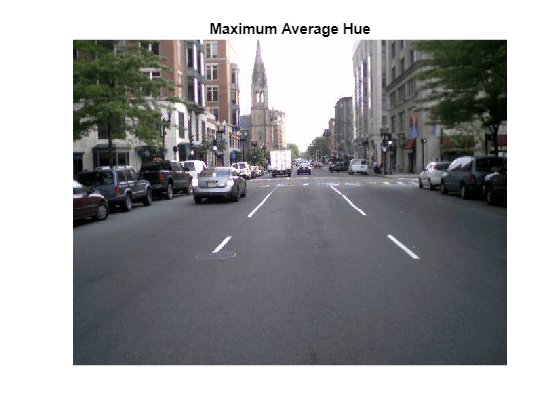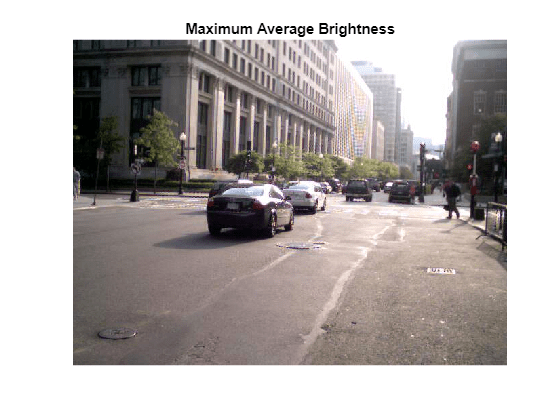# Read and Analyze Image Files

This example shows how to create a datastore for a collection of images, read the image files, and find the images with the maximum average hue, saturation, and brightness (HSV). For a similar example on image processing using the `mapreduce` function, see Compute Maximum Average HSV of Images with MapReduce.

Identify two MATLAB® directories and create a datastore containing images with `.jpg`, `.tif`, and `.png` extensions in those directories.

```location1 = fullfile(matlabroot,'toolbox','matlab','demos'); location2 = fullfile(matlabroot,'toolbox','matlab','imagesci'); ds = imageDatastore({location1,location2},'FileExtensions',{'.jpg','.tif','.png'});```

Initialize the maximum average HSV values and the corresponding image data.

```maxAvgH = 0; maxAvgS = 0; maxAvgV = 0; dataH = 0; dataS = 0; dataV = 0;```

For each image in the collection, read the image file and calculate the average HSV values across all of the image pixels. If an average value is larger than that of a previous image, then record it as the new maximum (`maxAvgH`, `maxAvgS`, or `maxAvgV`) and record the corresponding image data (`dataH`, `dataS`, or `dataV`).

```for i = 1:length(ds.Files) data = readimage(ds,i); % Read the ith image if ~ismatrix(data) % Only process 3-dimensional color data hsv = rgb2hsv(data); % Compute the HSV values from the RGB data h = hsv(:,:,1); % Extract the HSV values s = hsv(:,:,2); v = hsv(:,:,3); avgH = mean(h(:)); % Find the average HSV values across the image avgS = mean(s(:)); avgV = mean(v(:)); if avgH > maxAvgH % Check for new maximum average hue maxAvgH = avgH; dataH = data; end if avgS > maxAvgS % Check for new maximum average saturation maxAvgS = avgS; dataS = data; end if avgV > maxAvgV % Check for new maximum average brightness maxAvgV = avgV; dataV = data; end end end```

View the images with the largest average hue, saturation, and brightness.

```imshow(dataH,'InitialMagnification','fit'); title('Maximum Average Hue')``````figure imshow(dataS,'InitialMagnification','fit'); title('Maximum Average Saturation');``````figure imshow(dataV,'InitialMagnification','fit'); title('Maximum Average Brightness');```